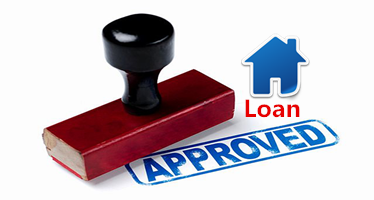# How much you worth to get loan from bank?

Before we start to invest in property, we need to know whether bank will approve our loan when we purchase some value of property. Therefore, how much you worth to get loan from bank is the key in property investment. You may need the help from loan officer to audit your current income and how much you worth?

Basically, for property loan application with bank, here are 3 important criteria:-

1). Purchase value of property

2). No of years for tenure

3). Loan interest rate

Total Loan Amount = Approved Loan @ 30 years (tenure years) / 6% (estimate loan interest rate)For loan amount that will approve by bank,

### Loan Amount = Total net income x 70% x (1000/6)

Example Case 1

Net income = RM 10K

Loan Amount = RM10k x 70% x (1000/6) = RM1,166,667

Example Case 2

Net income = RM 5K

Loan Amountt = RM5k x 70% x (1000/6) = RM 583,333

Example Case 3

Net income = RM 2K

Loan Amount = RM2k x 70% x (1000/6) = RM233,333

If 90% Loan,

### Value of Property You Can Buy = [ Total net income x 70% x (1000/6) ] / 90%

Example Case 1

Net income = RM 10K

Value of Property You Can Buy = [RM10k x 70% x (1000/6)]/ 90%

= RM1,296,297

Example Case 2

Net income = RM 5K

Value of Property You Can Buy= [RM5k x 70% x (1000/6)]/ 90%

= RM 648,148

Example Case 3

Net income = RM 2K

Value of Property You Can Buy= RM2k x 70% x (1000/6)

= RM259,259

Anyway, many people do not declare clearly the total income. Total income is not only your salary, it can be any source of income that you earn such as part time job income, rental income and any others income with proof of documents. Please maximize as possible you can!